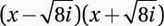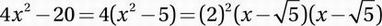# SAT Math Multiple Choice Question 644: Answer and Explanation

### Test Information

Question: 644

14. If the polynomial P(x) has factors of 12, (x - 5), and (x + 4), which of the following must also be a factor of P(x)?

• A. 2x2 + 8
• B. 4x2 - 20
• C. 6x2 - 6x - 120
• D. x2 - 10x + 25

Explanation:

C

Advanced Mathematics (analyzing polynomial functions) HARD

The simplest polynomial with factors of 12, (x - 5), and (x + 4) is P(x) = 12(x - 5)(x + 4). The completely factored form (including the prime factorization of the coefficient) of this polynomial is P(x) = (2)2 (3)(x - 5)(x + 4).

Now, using the methods we discussed in Chapter 9, Lesson 4, we can look at the factored form of each choice:

(A) 2x2 + 8 = 2(x2 + 8) (x2 + 8 is not factorable over the
reals, but it does equal(B)(C) 6x2 - 6x - 120 = 6(x2 - x - 20) = (2)(3)(x - 5)(x + 4)

(D) x2 - 10x + 25 = (x - 5)(x - 5)

Notice that every polynomial in (A), (B), and (D) contains at least one factor that is NOT in the factored form of P(x). (In (D), the factor (x - 5) appears twice, but it appears only once in P(x).) Only choice (C) contains ONLY factors that appear in P(x), so it is the only choice that must be a factor of P(x).